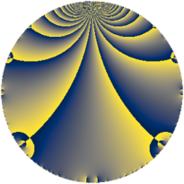# Properties

 Label 5520.2.a.bhLevel $5520$ Weight $2$ Character orbit 5520.a Self dual yes Analytic conductor $44.077$ Analytic rank $0$ Dimension $2$ CM no Inner twists $1$

# Learn more about

## Newspace parameters

 Level: $$N$$ $$=$$ $$5520 = 2^{4} \cdot 3 \cdot 5 \cdot 23$$ Weight: $$k$$ $$=$$ $$2$$ Character orbit: $$[\chi]$$ $$=$$ 5520.a (trivial)

## Newform invariants

 Self dual: yes Analytic conductor: $$44.0774219157$$ Analytic rank: $$0$$ Dimension: $$2$$ Coefficient field: $$\Q(\sqrt{17})$$ Defining polynomial: $$x^{2} - x - 4$$ Coefficient ring: $$\Z[a_1, \ldots, a_{7}]$$ Coefficient ring index: $$1$$ Twist minimal: no (minimal twist has level 2760) Fricke sign: $$-1$$ Sato-Tate group: $\mathrm{SU}(2)$

## $q$-expansion

Coefficients of the $$q$$-expansion are expressed in terms of $$\beta = \frac{1}{2}(1 + \sqrt{17})$$. We also show the integral $$q$$-expansion of the trace form.

 $$f(q)$$ $$=$$ $$q - q^{3} - q^{5} + \beta q^{7} + q^{9} +O(q^{10})$$ $$q - q^{3} - q^{5} + \beta q^{7} + q^{9} + 2 \beta q^{11} + 2 q^{13} + q^{15} + ( 2 + \beta ) q^{17} + ( 4 - 2 \beta ) q^{19} -\beta q^{21} + q^{23} + q^{25} - q^{27} + ( 6 + \beta ) q^{29} + ( -4 + 3 \beta ) q^{31} -2 \beta q^{33} -\beta q^{35} + ( -2 + \beta ) q^{37} -2 q^{39} + ( 6 - \beta ) q^{41} + ( -4 + 4 \beta ) q^{43} - q^{45} -8 q^{47} + ( -3 + \beta ) q^{49} + ( -2 - \beta ) q^{51} + ( -2 + \beta ) q^{53} -2 \beta q^{55} + ( -4 + 2 \beta ) q^{57} + ( -4 - \beta ) q^{59} + ( -2 + 6 \beta ) q^{61} + \beta q^{63} -2 q^{65} + \beta q^{67} - q^{69} + ( -4 - 3 \beta ) q^{71} + 2 q^{73} - q^{75} + ( 8 + 2 \beta ) q^{77} -4 \beta q^{79} + q^{81} + ( 8 - 3 \beta ) q^{83} + ( -2 - \beta ) q^{85} + ( -6 - \beta ) q^{87} + ( 2 - 2 \beta ) q^{89} + 2 \beta q^{91} + ( 4 - 3 \beta ) q^{93} + ( -4 + 2 \beta ) q^{95} -6 q^{97} + 2 \beta q^{99} +O(q^{100})$$ $$\operatorname{Tr}(f)(q)$$ $$=$$ $$2q - 2q^{3} - 2q^{5} + q^{7} + 2q^{9} + O(q^{10})$$ $$2q - 2q^{3} - 2q^{5} + q^{7} + 2q^{9} + 2q^{11} + 4q^{13} + 2q^{15} + 5q^{17} + 6q^{19} - q^{21} + 2q^{23} + 2q^{25} - 2q^{27} + 13q^{29} - 5q^{31} - 2q^{33} - q^{35} - 3q^{37} - 4q^{39} + 11q^{41} - 4q^{43} - 2q^{45} - 16q^{47} - 5q^{49} - 5q^{51} - 3q^{53} - 2q^{55} - 6q^{57} - 9q^{59} + 2q^{61} + q^{63} - 4q^{65} + q^{67} - 2q^{69} - 11q^{71} + 4q^{73} - 2q^{75} + 18q^{77} - 4q^{79} + 2q^{81} + 13q^{83} - 5q^{85} - 13q^{87} + 2q^{89} + 2q^{91} + 5q^{93} - 6q^{95} - 12q^{97} + 2q^{99} + O(q^{100})$$

## Embeddings

For each embedding $$\iota_m$$ of the coefficient field, the values $$\iota_m(a_n)$$ are shown below.

For more information on an embedded modular form you can click on its label.

Label $$\iota_m(\nu)$$ $$a_{2}$$ $$a_{3}$$ $$a_{4}$$ $$a_{5}$$ $$a_{6}$$ $$a_{7}$$ $$a_{8}$$ $$a_{9}$$ $$a_{10}$$
1.1
 −1.56155 2.56155
0 −1.00000 0 −1.00000 0 −1.56155 0 1.00000 0
1.2 0 −1.00000 0 −1.00000 0 2.56155 0 1.00000 0
 $$n$$: e.g. 2-40 or 990-1000 Significant digits: Format: Complex embeddings Normalized embeddings Satake parameters Satake angles

## Atkin-Lehner signs

$$p$$ Sign
$$2$$ $$1$$
$$3$$ $$1$$
$$5$$ $$1$$
$$23$$ $$-1$$

## Inner twists

This newform does not admit any (nontrivial) inner twists.

## Twists

By twisting character orbit
Char Parity Ord Mult Type Twist Min Dim
1.a even 1 1 trivial 5520.2.a.bh 2
4.b odd 2 1 2760.2.a.p 2
12.b even 2 1 8280.2.a.be 2

By twisted newform orbit
Twist Min Dim Char Parity Ord Mult Type
2760.2.a.p 2 4.b odd 2 1
5520.2.a.bh 2 1.a even 1 1 trivial
8280.2.a.be 2 12.b even 2 1

## Hecke kernels

This newform subspace can be constructed as the intersection of the kernels of the following linear operators acting on $$S_{2}^{\mathrm{new}}(\Gamma_0(5520))$$:

 $$T_{7}^{2} - T_{7} - 4$$ $$T_{11}^{2} - 2 T_{11} - 16$$ $$T_{13} - 2$$ $$T_{17}^{2} - 5 T_{17} + 2$$ $$T_{19}^{2} - 6 T_{19} - 8$$

## Hecke characteristic polynomials

$p$ $F_p(T)$
$2$ $$T^{2}$$
$3$ $$( 1 + T )^{2}$$
$5$ $$( 1 + T )^{2}$$
$7$ $$-4 - T + T^{2}$$
$11$ $$-16 - 2 T + T^{2}$$
$13$ $$( -2 + T )^{2}$$
$17$ $$2 - 5 T + T^{2}$$
$19$ $$-8 - 6 T + T^{2}$$
$23$ $$( -1 + T )^{2}$$
$29$ $$38 - 13 T + T^{2}$$
$31$ $$-32 + 5 T + T^{2}$$
$37$ $$-2 + 3 T + T^{2}$$
$41$ $$26 - 11 T + T^{2}$$
$43$ $$-64 + 4 T + T^{2}$$
$47$ $$( 8 + T )^{2}$$
$53$ $$-2 + 3 T + T^{2}$$
$59$ $$16 + 9 T + T^{2}$$
$61$ $$-152 - 2 T + T^{2}$$
$67$ $$-4 - T + T^{2}$$
$71$ $$-8 + 11 T + T^{2}$$
$73$ $$( -2 + T )^{2}$$
$79$ $$-64 + 4 T + T^{2}$$
$83$ $$4 - 13 T + T^{2}$$
$89$ $$-16 - 2 T + T^{2}$$
$97$ $$( 6 + T )^{2}$$
show more
show less